# The Cotangent Complex and Derived de Rham Cohomology

## Motivation

Basic affine objects in AG: commutative rings, replace with simplicial commutative rings which we’ll use as a base diagram. Later: derived stacks and geometric derived stacks. Here is an evolution of objects. and how we can think about them:

Algebraic schemes/spaces, e.g. $${\mathbb{P}}^n$$. Think of these as étale sheaves of sets (think functor of points), identified as discrete spaces: \begin{align*} \mathcal{S}_{\leq 0} \mathrel{\vcenter{:}}=\left\{{\text{Discrete spaces}}\right\} .\end{align*} Every component is contractible, so there are no higher homotopy groups and we think of these as 0-truncated spaces.

For $$X$$ a smooth and proper $$k{\hbox{-}}$$scheme, the Picard stack \begin{align*} \underline{{\operatorname{Pic}}}_{X/k} \end{align*} is an Artin stack, which is a subclass Deligne-Mumford stacks. Note that this still has automorphisms given by global units on $$X$$. Think of these as \begin{align*} \mathcal{S}_{\leq 1} \mathrel{\vcenter{:}}=\left\{{\text{Étale sheaves of groupoids}}\right\} ,\end{align*} where the notation now suggests 1-truncated spaces, and we can take fundamental groupoids $$\Pi_0$$ since there is now 1-homotopy.

Note that the Picard stack can be identified as a mapping stack, \begin{align*} \underline{{\operatorname{Map}}}(X, K({\mathbb{G}}_m, 1)) .\end{align*}

$$K({\mathbb{G}}_m, n)$$ is a “higher stack,” thought of as a sheaf taking values in $$n{\hbox{-}}$$truncated spaces $$\mathcal{S}_{\leq n}$$, i.e. a space where when basing at any point, there are no homotopy groups above degree $$n$$: \begin{align*} \mathcal{S}_{\leq n} \mathrel{\vcenter{:}}=\left\{{\text{Étale sheaves of n{\hbox{-}}truncated spaces}}\right\} .\end{align*} This is a stack with a single point, where the isotopy is $$K({\mathbb{G}}_m, n-1)$$.

Note that these are all built from affine schemes with a few acceptable moves.

(DZG) The definitions of $$\mathcal{S}_{j}$$ above aren’t explicitly stated, so these are guesses at slightly more precise definitions.

In stack notation, we can write \begin{align*} B{\mathbb{G}}_m &= K({\mathbb{G}}_m, 1) \cong [{\{\text{pt}\}}/{\mathbb{G}}_m] \\ K({\mathbb{G}}_m, 2) &= [{\{\text{pt}\}}/ B{\mathbb{G}}_m] ,\end{align*} where the latter is a smooth Artin stack. Mapping into this gives the Picard groupoid of a scheme. These are higher geometric stacks that still have some “smoothness” properties.

What does it mean to give a map from a scheme $$X$$ into a higher stack?

Note that the category of étale schemes taking values in $$\mathcal{S}_{\leq n}$$ is enriched in topological spaces. There is a topological space \begin{align*} M\mathrel{\vcenter{:}}={\operatorname{Map}}(X, K({\mathbb{G}}_m, n)) \end{align*} where the homotopy groups are \begin{align*} \pi_i M = \begin{cases} H^{n-i}_{\mathrm{\text{ét}}}(X, {\mathbb{G}}_m) & 0\leq i \leq n \\ 0 & \text{else} \end{cases} .\end{align*} So this higher geometric stack that says something about higher étale cohomology groups. We thus have étale sheaves taking values in higher topological spaces, and has some geometric meaning. They’re also built from geometric objects: namely, iterating taking quotients by smooth actions. $$K({\mathbb{G}}_m, 1)$$ is a quotient by a smooth algebraic group, $$K({\mathbb{G}}_m, 2)$$ is now a smooth Artin stack, and we can keep going. This is the fundamental process for building geometric higher stacks.

Why derive things? Schemes are equipped with sheaves of commutative rings, so the basic idea is let the sheaves take values in groupoids, stacks, etc. So we can consider replacing the structure sheaf $${\mathcal{O}}_X$$ is itself a sheaf of spaces, and this is the fundamental idea of derived algebraic geometry.

## Derived Schemes

Consider $$\operatorname{Spec}k\otimes_{k[x]}^L k$$, a derived tensor product. This is a simplicial commutative ring, and the basic version of an affine derived scheme. This is a complex $$C^{\,\cdot\,}$$ with homology in degree 0 and 1, where \begin{align*} H_1 = \text{Tor}^1(k\otimes_{k[x]} k) \end{align*} So analogously, we’ll start with derived schemes and take quotients by smooth groups. In the end, we get derived stacks.

An example is $$\mathcal{M}_\phi$$, the moduli of objects in some DG category $$\mathcal{C}$$.

## Simplicial Rings

We need to agree on what the local affine modules will look like. For our purposes, they’ll be simplicial commutative rings. Consider the derived category $$\mathcal{C} \mathrel{\vcenter{:}}= D({\mathbb{Z}})_{\geq 0}$$ and its connective1 objects, which are chain complexes $$C_{\,\cdot\,}$$ where $$H_{< 0}(C_{\,\cdot\,}) = 0$$. There is a derived tensor product $$\otimes^L$$ which makes $$\mathcal{C}$$ into a symmetric monoidal category.

Basic idea: we want to look at commutative algebra objects in this symmetric monoidal category $$({D}({\mathbb{Z}}), \otimes^L)$$.2 Note that of working in a symmetric monoidal abelian category, we will be looking at connective chain complexes, and simplicial rings are one way of studying commutative algebra objects here.

We have some choices for making sense of DAG:

• $$E_\infty{\hbox{-}}$$ring spectra

• Simplicial commutative rings.

• Over $${\mathbb{Q}}$$, $${\mathbb{Q}}{\hbox{-}}$$commutative DGAs.

Our choice here will be the following:

Let $$\Delta$$ denote the simplex category, the category of non-empty finite ordered sets with order-preserving maps. We have the following situation:The arrows going up are face maps (or coface maps), and the others are degeneracy maps. If $$\mathcal{C}$$ is a category, then $$s\mathcal{C} \mathrel{\vcenter{:}}={\text{Fun}}(\Delta^\text{op}, \mathcal{C})$$ is the category of simplicial objects of $$\mathcal{C}$$.

An analogy: simplicial commutative $$k{\hbox{-}}$$algebras enrich usual $$k{\hbox{-}}$$algebras much like the derived category $$D(k)$$ enriches $$k{\hbox{-}}$$modules.

## Examples of Simplicial Categories

### a: Simplicial Sets and Topological Spaces

$$\operatorname{sSets}\simeq{\text{Top}}$$: this is not an equivalence of categories, but rather they have equivalent homotopy theories3, where we have notions of weak equivalence4 on each side. Here there is an $$n{\hbox{-}}$$simplex on the LHS ($$\operatorname{sSets}$$), \begin{align*} \Delta^n = \hom_{\Delta}({\,\cdot\,}, [n]) \end{align*} and on the RHS we have \begin{align*} \Delta^n_{\text{Top}}\mathrel{\vcenter{:}}=\left\{{{\left[ {x_0, \cdots, x_n} \right]} \in {\mathbb{R}}^n ~{\text{s.t.}}~x_i\geq 0,\, \sum x_i = 1}\right\} \end{align*}

If you make a functor $$\Delta^n \rightleftharpoons\Delta^n_{\text{Top}}$$, then by Yoneda the presheaf category $${\operatorname{Presh}}(\Delta) \mathrel{\vcenter{:}}={\text{Fun}}(\Delta^\text{op}, {\text{Set}})$$ is generated by representable objects. Everything on in $$\operatorname{sSets}$$ is generated by taking colimits of the $$\Delta^n$$, so we can make some assignment and extend by colimits to get a functor $$\operatorname{sSets}\to {\text{Top}}$$. We have a notion of weak equivalence for $${\text{Top}}$$, and so the notion of weak equivalence on $$\operatorname{sSets}$$ is just given by pullback along the functor $$\operatorname{sSets}\to {\text{Top}}$$, and this induces an equivalence of homotopy theories. The inverse functor $${\text{Top}}\to \operatorname{sSets}$$ is the singular complex construction. Considering $$\Delta^{\,\cdot\,}_{{\text{Top}}}$$, this is a cosimplicial object in $${\text{Top}}$$.

$${\text{Top}}$$ will denote that 1-category, while $$\mathcal{T}\text{op}$$ will be its full $$\infty{\hbox{-}}$$category.

So we have a natural cosimplicial object in $${\text{Top}}$$, so \begin{align*} \text{Sing}(X) \mathrel{\vcenter{:}}={\operatorname{Hom}}_{{\text{Top}}}(\Delta_{{\text{Top}}}^{\,\cdot\,}, X) \end{align*} is a simplicial object in $$\operatorname{sSets}$$. As in singular homology, we can get a simplicial abelian group by taking the free abelian group $${\mathbb{Z}}[{\text{Sing}}(X)]$$. Note that this is just composing functors $$\Delta^\text{op}\to{\text{Set}}$$ and $${\text{Set}}\to {{{\mathbb{Z}}}{\hbox{-}}\operatorname{mod}}$$. We can use this to create a chain complex $$C_{\,\cdot\,}({\mathbb{Z}}[\text{Sing}(X)])$$, and as expected, we get the singular homology: \begin{align*} H_i(C_{\,\cdot\,}) \cong H^{\text{Sing}}_i(X, {\mathbb{Z}}) \end{align*}

### b: Simplicial Abelian Groups

We can take simplicial abelian groups $$s{\text{Ab}}$$ and the connective objects $$D({\mathbb{Z}})_{\geq 0}$$; these have equivalent homotopy theories. There is a notion of weak equivalence on the RHS, quasi-isomorphism, and asking if the literal underlying spaces on the LHS are weakly equivalence as spaces. A specific way of doing this is the Dold-Kan correspondence:

Suppose we have a simplicial abelian group $$M_{\,\cdot\,}$$, then we have face maps going to the left: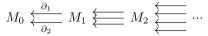We make this into a chain complex by setting the differential to a graded sum: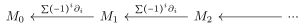The homology of this complex turns out to be the same as the homotopy groups of the simplicial abelian group viewed as a topological space.

### c: Simplicial Commutative $$k{\hbox{-}}$$Algebras

Defined as \begin{align*} s\mathrm{CAlg}_k \mathrel{\vcenter{:}}={\text{Fun}}(\Delta^\text{op},\mathrm{CAlg}_k) ,\end{align*} where $$k$$ is some commutative ring. This was studied by Quillen, and an impetus for model categories. Models give a notion of weak equivalence and a “right way” of computing: for the usual derived category of a ring, this is taking free/projective/injective resolutions. So the LHS is sometimes called a non-abelian derived category.

For $$R\in \mathrm{sCAlg}$$, the homotopy groups $$\pi_* R$$ have a graded commutative ring structure: $$xy = (-1)^{{\left\lvert {x} \right\rvert} {\left\lvert {y} \right\rvert}}yx$$ and $$x^2 =0$$ for elements $$x$$ with $${\left\lvert {x} \right\rvert}$$ odd. This is useful because it gives us some graded ring to associated to $$R$$.

The category of simplicial abelian groups is equivalent to $$\mathrm{Ch}({\mathbb{Z}})_{\geq 0}$$; i.e. chain complexes of abelian groups concentrated in non-negative degree. This also yields an equivalence of homotopy theories.

A different perspective on simplicial commutative rings: there is an adjunction from sets to commutative $$k{\hbox{-}}$$algebras

\begin{align*} {\text{Set}}&\rightleftharpoons{}{} \mathrm{CAlg}_k \\ S &\mapsto K[S] .\end{align*} i.e. we send a set to the polynomial ring generated by $$S$$. Any time such an adjunction exists, given an $$R\in \mathrm{CAlg}_k$$ we can construct a simplicial resolution $$S^{\,\cdot\,}$$ and a map $$S^{\,\cdot\,}\to R$$. This resolution has the following structure: \begin{align*} S^0 = k[R], \,\, \text{the free commutative algebra on }R .\end{align*} Using the unit and counit maps of the adjunction, one obtains a canonical simplicial object,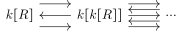Moreover, $$S^{\,\cdot\,}\xrightarrow{\sim} R$$ is a homotopy equivalence. So we’ve taken an arbitrary $$k{\hbox{-}}$$algebra and replaced it with a simplicial $$k{\hbox{-}}$$algebra which is given by polynomial rings in each degree, typically in infinitely many variables, which has the same homology. This is the analog of a projective resolution.

Now define $$\mathrm{CAlg}_k^{\text{poly}}$$ as the category of finitely generated polynomial rings, and suppose you have a functor \begin{align*} \mathrm{CAlg}_k^{\text{poly}} \xrightarrow{F} \mathcal{C} \end{align*} where $$\mathcal{C}$$ is a “reasonable” category or possible in $$\infty{\hbox{-}}$$category. We can consider the category $$\operatorname{Ind}(\mathrm{CAlg}_k^{\text{poly}})$$ given by formally adjoining filtered colimits. We have the following diagram,where the bottom inclusion is given by viewing a commutative ring as the constant simplicial commutative ring, and the extension $$\tilde F$$ exists by applying $$F$$ to any colimit diagram. The functor $$LF$$ is a derived functor that exists if $$\mathcal{C}$$ has certain colimits.

So starting with a functor defined on finitely generated polynomial rings, i.e. affine spaces, we get a simplicial derived functor. For $$R\in \mathrm{CAlg}_k$$, using $$S^{\,\cdot\,}\xrightarrow{\sim} R$$, we can apply $$F$$ level-wise to get a new simplicial object $$F(S^{\,\cdot\,}) \in \mathcal{C}$$. Then $$LF(R)$$ is defined by taking the colimit over $$\Delta$$ yields the geometric realization, i.e.  \begin{align*} LF(R) \mathrel{\vcenter{:}}=\mathop{\mathrm{hocolim}}_{\Delta} F(S^{\,\cdot\,}) = {\left\lvert {F(S^{\,\cdot\,})} \right\rvert} \end{align*} So we can promote functors on polynomial rings to functors on simplicial commutative rings. This ends up being a Kan extension. There is a nice universal property here, namely that functors out of $$\mathrm{sAlg}_k$$ are equivalent to functors out of $$\mathrm{CAlg}_k^{\text{poly}}$$ that satisfy some additional properties.

The last example will be the cotangent complex.

## The Cotangent Complex

There are 3 equivalent ways to view the cotangent complex.

### 1: A Derived Functor Approach

Use this derived functor approach: it will essentially be the derived functor of taking $$\Omega^1$$. Suppose $$k \to R$$ in commutative rings, denote by $$S^{\,\cdot\,}$$ again the canonical resolution of $$R$$, yielding a diagram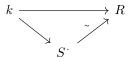Take Kahler differentials degree-wise to get $$\Omega^1_{S^{\,\cdot\,}/k}$$ . Now base-change along this map to get a simplicial $$R{\hbox{-}}$$module, \begin{align*} \Omega^1_{S^{\,\cdot\,}/k} \otimes_{S^{\,\cdot\,}} R \,\, \in \mathrm{sMod}{\hbox{-}}R .\end{align*} We can now use Dold-Kan to view this as a connective object in $$D(R)_{\geq 0}$$, the derived category of $$R$$, yielding $$\mathrm{sMod}{\hbox{-}}R \simeq D(R)_{\geq 0}$$, so we define the cotangent complex of $$R/k$$ as \begin{align*} L_{R/k} \mathrel{\vcenter{:}}=\Omega^1_{S^{\,\cdot\,}/k} \otimes_{S^{\,\cdot\,}} R \,\, \in D(R)_{\geq 0} .\end{align*}

This turns out to work if you take a resolution other than the canonical one. A downside to this definition is that it’s not clear how/why it might depend on the resolution.

### 2: Extending Functors

We can define them by taking a map \begin{align*} \mathrm{CAlg}_k^{\text{poly}} \xrightarrow{\Omega^1_{{\,\cdot\,}/k}} D(k)_{\geq 0} \end{align*} to the connective objects in the derived category of $$k$$. We can extend to get a diagramIt then turns out that $$L_{R/k} \simeq L\Omega^1_{R/k}$$. Here it’s not quite clear why this lands in $${{k}{\hbox{-}}\operatorname{mod}}$$ instead of $${{R}{\hbox{-}}\operatorname{mod}}$$, or how the $$R{\hbox{-}}$$module structure works here.

### 3: A Universal Property

Let $$k\to R\to S$$ where $$k$$ is a commutative ring and $$R, S$$ are ordinary (or simplicial) commutative rings. Let $$M\in {{S}{\hbox{-}}\operatorname{mod}}$$ in $$D(S)_{\geq 0}$$. There is a natural enrichment in topological spaces, so we’ll write $${\operatorname{Map}}$$ for homs with their topological structure. In particular, these have homotopy groups. The universal property that the cotangent complex has comes from an equivalence \begin{align*} {\operatorname{Map}}_R(L_{R/k}, M) \simeq {\operatorname{Map}}_{\mathrm{sCAlg}_{k//S}}(R, S\oplus M) .\end{align*} where the latter is in the category of simplicial commutative $$k{\hbox{-}}$$algebras with a fixed map to $$S$$, a bit like a comma category. This makes sense because $$R\to S$$ is a fixed map, and $$S\oplus M$$ has a projection to $$S$$, i.e. this is a square zero extension.

The point of simplicial commutative rings is that this extension still makes sense, even when $$M$$ is a chain complex. It’s still possible to make this into a simplicial commutative ring. This may look familiar from the exercises in Hartshorne, since it resembles the definition of Kahler differentials.

#### Exercises Using the Universal Property

Prove that \begin{align*} \pi_0 L_{R/k} \cong \Omega^1_{R/k} .\end{align*} This is exactly lifting through the square zero extension. This is similar to having a lift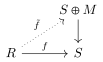where giving $$\tilde f$$ is like giving a derivation $$R\to M$$. So the RHS comma category mapping space in the previous equivalence is denoted the “space of derivations of $$R$$ and $$M$$” (whatever that means)

You can base-change the cotangent complex of $$R/K$$ to $$S$$ and obtain an exact triangle/cofiber sequence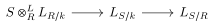This follows from the universal property above, and is a form of “transitivity.”

Given a diagramshow that \begin{align*} T\otimes_k^L L_{R/k} \cong L_{R\otimes_k^L T / T} .\end{align*}

All of these follow from just the mapping space property, but require thinking about what it means to compute maps in a comma category.

Let $$R$$ be a perfect $${\mathbb{F}}_p{\hbox{-}}$$algebra, so the Frobenius is an isomorphism: \begin{align*} F: R &\xrightarrow{\sim} R\\ x &\mapsto x^p .\end{align*} Show that $$L_{R/{\mathbb{F}}_p} \cong 0$$.

## Derived de Rham Cohomology

We can make a specific functor: \begin{align*} \mathrm{CAlg}_k^{\text{poly}} &\to D(k)\\ R &\mapsto \mathrm{dR}_{R/k} \mathrel{\vcenter{:}}=\qty{R\to \Omega^1_{R/k} \to \Omega^2_{R/k} \to \cdots} ,\end{align*} which takes a polynomial ring $$R$$ to its algebraic de Rham complex. If $$R$$ is finitely generated, then the complex is bounded. One can formally extend by deriving this functor to obtainThe point is that we know de Rham cohomology behaves well for smooth things, and we want to extend to non-smooth things.

Suppose $$k \in \mathrm{Alg}_{\mathbb{Q}}$$, noting that we’re in characteristic zero, and let $$R \in \mathrm{CAlg}_k^\text{poly}$$ be a polynomial ring. Then $$\mathrm{dR}_{R/k} \cong k$$ by the Poincaré lemma, just the cohomology of affine space. Then $$L\mathrm{dR}_{S/k} \cong k$$, which is disappointing.

### The Hodge Filtration

How to fix this: we have the Hodge filtration $$F_H^{\,\cdot\,}\mathrm{dR}_{R/k}$$, and the graded pieces are given by \begin{align*} {\operatorname{gr}}^i_H \mathrm{dR}_{R/k} \cong \Omega^i_{R/k}[-i] \cong \Lambda^i \Omega^1_{R/k} ,\end{align*} a shifted version of the $$i$$th exterior power 1-forms. Taking these gradings is compatible with colimits, so we can remember the filtration in the Kan extension, yielding a canonical Hodge filtration on $$L\mathrm{dR}$$, $$F^*_H L \mathrm{dR}_{{\,\cdot\,}/k}$$. The graded pieces are given by \begin{align*} {\operatorname{gr}}^i_H L\mathrm{dR}_{{\,\cdot\,}/k} \cong L \Lambda^i L_{R/k}[-i] ,\end{align*} i.e. you take derived exterior powers of the cotangent complex, shifted by some degrees. This follows because doing the Kan extension on the graded pieces is deriving the functor $${\operatorname{gr}}^i_H \mathrm{dR}_{{\,\cdot\,}/k}$$. We can complete with respect to this filtration, which we’ll write has

\begin{align*} \widehat{L\mathrm{dR}_{{\,\cdot\,}/k}} \mathrel{\vcenter{:}}=\varprojlim L\mathrm{dR}_{{\,\cdot\,}/k} / F_H^i ,\end{align*} where you quotient out by the $$i$$th piece of the filtration. Note that this definition is a general way of taking completions.5

Suppose $$X/{\mathbb{C}}$$ is finite type, then \begin{align*} R \Gamma(X, \widehat{ L\mathrm{dR}_{{\mathcal{O}}_X/ k} } ) \cong R \Gamma_{{\text{Sing}}}(X({\mathbb{C}}), {\mathbb{C}}) ,\end{align*} where the RHS is the singular cohomology of the $${\mathbb{C}}{\hbox{-}}$$points. This is a generalization of Grothendieck’s theorem in the smooth case. A positive feature is that this doesn’t depend on a choice of putting $$X$$ in an ambient smooth scheme.

1. Connective means $$H_{<0} = 0$$.↩︎

2. This is a familiar move: people in the 60s knew one could do AG in some ambient symmetric monoidal abelian category.↩︎

3. Theory up to weak equivalence.↩︎

4. A weak equivalence is an isomorphism on $$\pi_0$$, and for each choice of basepoint, an isomorphism on all $$\pi_{\geq 1}$$ on each side.↩︎

5. This is like defining the $$p{\hbox{-}}$$adics as the limit of $${\mathbb{Z}}/p^n{\mathbb{Z}}$$.↩︎